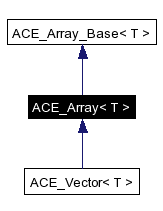# ACE_Array< T > Class Template Reference

A dynamic array class. More...

`#include <Containers_T.h>`

Inheritance diagram for ACE_Array< T >:[legend]
Collaboration diagram for ACE_Array< T >:[legend]
List of all members.

## Public Types

typedef T TYPE
typedef ACE_Array_Iterator<
T >
ITERATOR

## Public Member Functions

ACE_Array (size_t size=0, ACE_Allocator *alloc=0)
Dynamically create an uninitialized array.
ACE_Array (size_t size, const T &default_value, ACE_Allocator *alloc=0)
Dynamically initialize the entire array to the {default_value}.
ACE_Array (const ACE_Array< T > &s)
Copy constructor.
void operator= (const ACE_Array< T > &s)
Assignment operator.
bool operator== (const ACE_Array< T > &s) const
Equality comparison operator.
bool operator!= (const ACE_Array< T > &s) const
Inequality comparison operator.

## Detailed Description

### template<class T> class ACE_Array< T >

A dynamic array class.

This class extends ACE_Array_Base, adding comparison operators.

Requirements and Performance Characteristics

• Internal Structure Dynamic array
• Duplicates allowed? Yes
• Random access allowed? Yes
• Search speed N/A
• Insert/replace speed O(1)
• Iterator still valid after change to container?
• In general, yes.
• If array size is changed during iteration, no.
• Frees memory for removed elements? No
• Items inserted by Value
• Requirements for contained type
1. Default constructor
2. Copy constructor
3. operator=
4. operator!=

ACE_Array_Base. This class inherits its operations and requirements.

## Member Typedef Documentation

 template typedef ACE_Array_Iterator ACE_Array< T >::ITERATOR
 Reimplemented from ACE_Array_Base< T >.

 template typedef T ACE_Array< T >::TYPE
 Reimplemented from ACE_Array_Base< T >.

## Constructor & Destructor Documentation

 template ACE_INLINE ACE_Array< T >::ACE_Array ( size_t size = `0`, ACE_Allocator * alloc = `0` )
 Dynamically create an uninitialized array. Initialize an empty array of the specified size using the provided allocation strategy.

 template ACE_INLINE ACE_Array< T >::ACE_Array ( size_t size, const T & default_value, ACE_Allocator * alloc = `0` )
 Dynamically initialize the entire array to the {default_value}. Initialize an array the given size placing the default_value in each index.

 template ACE_INLINE ACE_Array< T >::ACE_Array ( const ACE_Array< T > & s )
 Copy constructor. The copy constructor performs initialization by making an exact copy of the contents of parameter {s}, i.e., *this == s will return true.

## Member Function Documentation

 template ACE_INLINE bool ACE_Array< T >::operator!= ( const ACE_Array< T > & s ) const
 Inequality comparison operator. Compare this array with {s} for inequality such that {*this} != {s} is always the complement of the boolean return value of {*this} == {s}.

 template ACE_INLINE void ACE_Array< T >::operator= ( const ACE_Array< T > & s )
 Assignment operator. Assignment operator performs an assignment by making an exact copy of the contents of parameter {s}, i.e., *this == s will return true. Note that if the {max_size_} of {array_} is >= than {s.max_size_} we can copy it without reallocating. However, if {max_size_} is < {s.max_size_} we must delete the {array_}, reallocate a new {array_}, and then copy the contents of {s}.

 template bool ACE_Array< T >::operator== ( const ACE_Array< T > & s ) const
 Equality comparison operator. Compare this array with {s} for equality. Two arrays are equal if their {size}'s are equal and all the elements from 0 .. {size} are equal.

The documentation for this class was generated from the following files:
Generated on Sun Mar 5 15:39:10 2006 for ACE by1.3.9.1